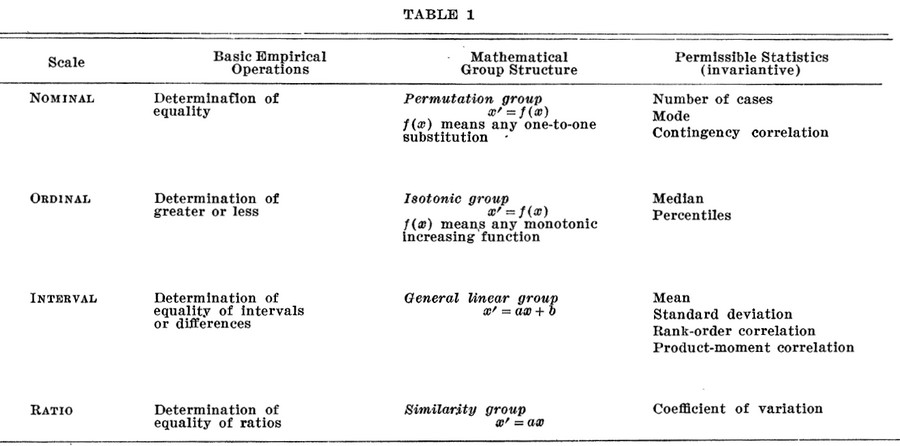# Statistics - (Interval|Delta) (Measurement)

An interval (measurement|variable) gives:

## Properties

The following properties applied to interval measurement:

• The interval between each unit are equals (temperature between 21 and 22 degree is the same than between 22 and 23 degree)
• There is no absolute (true zero) when interval are scaled.

## Example

• longitude and latitude
• temperature (either Celsius or Fahrenheit degrees). A change of 1C is the same if the temperature goes from 0 C to 1 C, as it is when the temperature goes from 40C to 41C.
• time,
• distance,
• area
• volume

## Documentation / Reference

Discover MoreDistance

is a numerical description of how far apart objects are. Same as: In most cases, “distance from A to B” is interchangeable with “distance between B and A”. In physics or everyday...Process - Poisson Process

The Poisson process is a stochastic process in which events occur: continuously independently (of the time since the last event) - (ie random) at a constant / known average rate in a fixed interval...Statistics - (Fraction|Ratio|Percentage|Share) (Variable|Measurement)

Ratio measurement gets its name from the fact that meaningful fraction (ratio or rational number) can be constructed with a ratio variable. Ratio is the deepest, most meaningful level of measurement,...Statistics - (Scales of measurement|Type of variables)

According to Stevens theory (On the Theory of Scales of Measurement), the four scales of measurement are: nominal ordinal ...Statistics - Deviation Score (for one observation)

The deviation score is the difference between two scores as : a value and an other value (usually the predicted score: mean, ...) See also: for a sample, for a measurement, (??) ...Statistics - Exponential Distribution

exponential in Distribution exponential distribution is the probability distribution of the time between events in a Poisson process. The value (mostly time) between each pair of consecutive events in...Statistics - Poisson Distribution

The Poisson distribution is a discrete probability distribution (of count) that expresses the probability of a given number of events (count) that will occur in an interval on a Poisson process Like the...